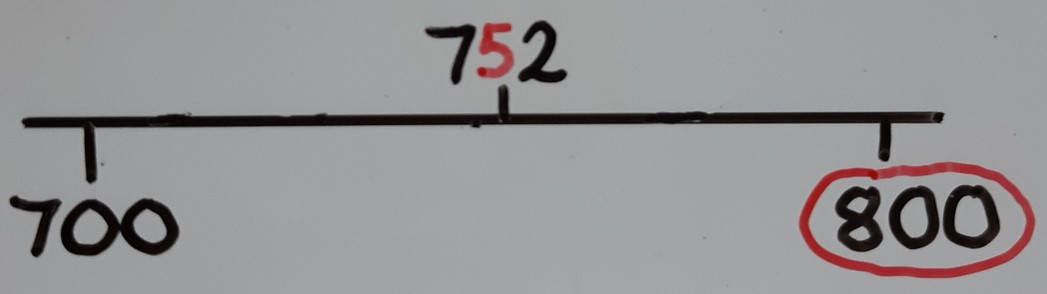## Rounding to the nearest 100

When rounding to the nearest 100, the process is similar to rounding to the nearest 10, only this time we are checking which multiple of 100 the number is closest to. Pupils first need to practise placing numbers with 3 or more digits correctly on a numberline marked in multiples of 100, to identify the halfway numbers and recognise that they always have 5 tens. This leads to understanding that it is necessary to check the tens digit to see which multiple of 100 it is closest to. The ones digit is ignored, regardless of its size.713 has less than 5 tens, meaning it is closer to 700 than 800, so it rounds down to 700.781 has more than 5 tens, meaning it is closer to 800 than 700, so it rounds up to 800.752 has 5 tens, meaning it is exactly halfway between multiples, so it also rounds up to the next multiple of 100.### Summary

When rounding to the nearest 100 we check the tens digit:

If the tens digit is less than 5, we round down to the previous multiple of 100. The hundreds digit stays the same and we replace any tens/ones with zeroes.

If the tens digit is 5 or more, we round up to the next multiple of 100. We add 1 to the hundreds digit and we replace any tens/ones with zeroes.

After rounding to 100, the number will always end in at least two zeroes.

Play Rounding race to practice rounding to the nearest 100.